The ISP Column An occasional column on things Internet Other Formats: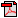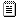Mutterings on MTUs
February 2009

Geoff Huston

In the previous column I explored an error I had encountered where an IPv4-only web browser on my dual stack system could connect to a web server and retrieve the web pages, while a dual stack configured browser managed to get itself stuck displaying a white page.

One possible response is to use active probing as a means of path MTU discovery, as described in RFC4821. Here the sending stack explicitly probes the path MTU using a path probe mechanism where the TCP stack periodically probes the path with a packet that is larger than the current path MTU estimate and a longer than usual associated ACK timer. Successful acknowledgement of the probe packet raises the path MTU estimate to the probe packet size, while the absence of an acknowledgement is treated as a probe failure, rather than a congestion indication, so that the TCP session does not throttle its sending rate in response to an MTU probe failure.

But packet probing as a means of Path MTU Discovery is not a common feature of currently deployed IPv6 implementations in end host stacks, so other approaches need to be considered. The previous column looked at a number of approaches that could work around the problem, but the basic question of path MTU management remains.

The logical question is: If fragmentation is causing such a problem then shouldn't we try to avoid the problem completely and just use the minimum packet size for all IP packets? After all, fragmentation is problematical for firewalls and filters, because fragments do not contain the TCP or UDP port addresses that are a conventional part of so many filtering roles, and fragmentation is a problem for the destination host in so far as each fragment that has a new IP identifier causes the destination to open up a new fragmentation reassembly context.

I offered the opinion that for IPv6 servers and clients, the conservative message if you want a robust service your best option is to set the server's MTU for IPv6 to at least 40 octets less than the interface MTU size, or even to consider setting this MTU value down to 1280 octets, the minimum universally supported non-fragmented IPv6 packet size, to take the most conservative position.

The conventional belief in this topic appears to be that the most compelling argument to raise the packet size above the minimum level is to maximise data performance. So it was not unexpected to see some comments in response that questioned the implicit compromise in performance through using a smaller MTU. One view was the drop dropping the MTU size just "cripples their own network". There appears to be a body of opinion that it's a more productive use of our time and effort to try and hunt down each and every point in the network where these ICMPv6 packet-too-big messages are either not being correctly generated, or are being blocked than to spend the time performing a configuration change of IPv6 servers to operate with an MTU size positioned at between 1400 to 1460 octets, or at least 40 octets less than the server's interface MTU size.

### The MTU Question

"Conventional beliefs" always intrigue me, in that while sometimes these beliefs express basic constraints and truths, at other times they are misleading and just plain wrong. So the question I'd like to look at in this article is: How bad is an MTU setting of 1280, as compared to an MTU setting of 1500? What performance differential can one expect and why? Is this really "crippling" to a network and its clients? What is the relationship between internet performance and the maximum packet size as set of the MTU setting?

The first, and perhaps the most obvious difference in MTU settings is the different relative amount of overhead from the packet and frame headers. For a TCP stream operating with the timestamp option set (which appears to be a typical setting for Apache web servers) the overhead is 20 octets of IPv4 header, or 40 octets of IPv6 header, 20 octets of TCP header and 12 octets of timestamp TCP option header, or a total of 52 or 72 octets. If an 802.3 Ethernet frame is being used there is a further framing overhead of 8 octets of preamble and start of frame delimiter, 12 octets of MAC addresses, 2 octets of Ethertype, and a trailing frame overhead of 4 octets of the CRC value and 12 octets of the interframe gap (Figure 1).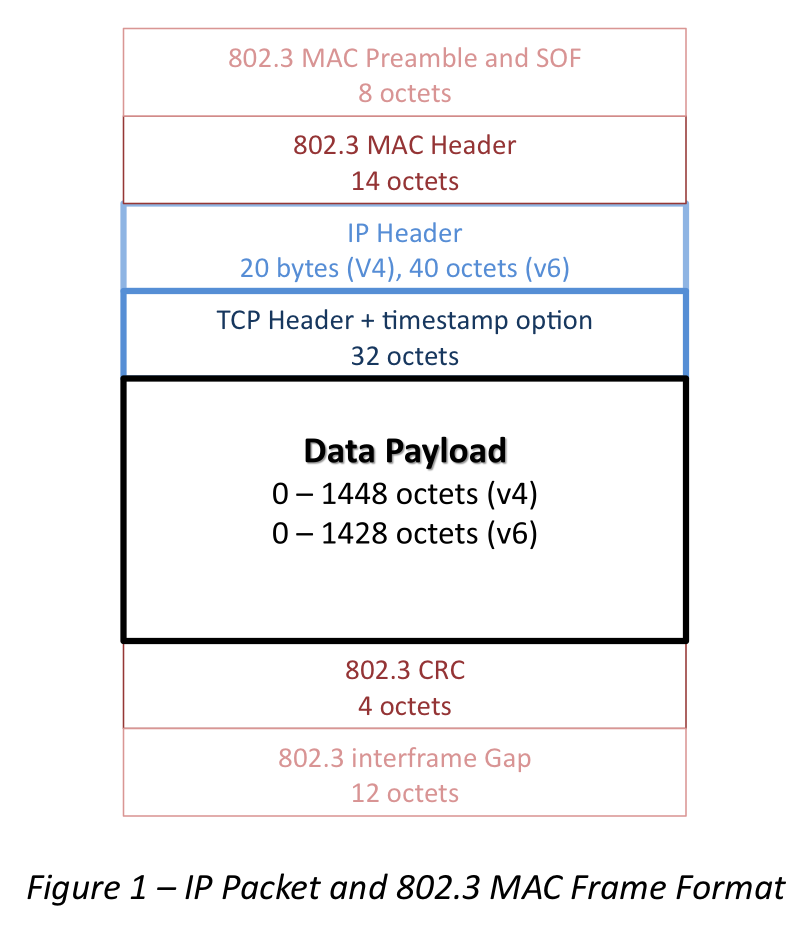Given that the per-packet overheads are of constant size, then the smaller the MTU the higher the relative overhead of the packet overheads. The following table shows the maximal TCP carriage efficiency when using a maximum-sized TCP packet stream using the TCP timestamp option and 802.3 MAC level framing.

 MTU Size IPv4 Payload 802.3 DataEfficiency IPv6 Payload 802.3 Data 9000 8948 99.00% 8928 98.78% 4000 3948 97.77% 3928 97.28% 1500 1448 94.15% 1428 92.85% 1480 1408 92.75% 1460 1388 92.66% 1400 1348 93.74% 1328 92.35% 1280 1228 93.17% 1208 91.65% 1000 948 91.33% 576 524 85.34%

One way to interpret this table is that, for example, the maximal data rate of a 100Mbps connection using IPv4 and a 1500 octet MTU is 94.15Mbps, while the maximal rate on the same 100Mbps Ethernet using an IPv6 connection is 92.85Mbps. If the IPv6 connection is tunneled using an IPv6 in IPv4 tunneling mechanism the IPv6 payload is reduced by a further 20 octets, bringing the maximal data throughput to 92.75%, and the use of a UDP tunnel, such as used by Teredo tunneling would bring this down further to 92.66Mbps.

In the previous column on MTU issues with IPv6 I suggested using a 1400 MTU as a conservative approach to avoid the issues associated with Path MTU discovery black holes. As shown in the above table, the overall impact of this approach of dropping the interface MTU from 1500 to 1400 octets for IPv6 is a net drop in the maximum attainable performance level of 92.85Mbps to 92.35Mbps for data throughput.

### TCP Performance and MTU size

When talking about flow performance in the Internet we are normally talking about the performance of the TCP protocol stack and the flow performance of a reliable data transfer as managed by a TCP rate control protocol. In this case I'm trying to look at the assessment of what it means to vary the MTU size in a TCP environment.

TCP is a rate adaptive control protocol where the sender is attempting to operate the connection at the maximum speed that is permitted by the available resources on the sending and receiving end host and to operate at a speed that represents a fair proportion of the available network path's resources.

The general characteristics of TCP rate control is shown in the following figure. TCP initially starts in a mode termed "Slow Start", and then may either stabilize at a resource-limited sending rate, or use a dynamic rate control algorithm termed "congestion avoidance".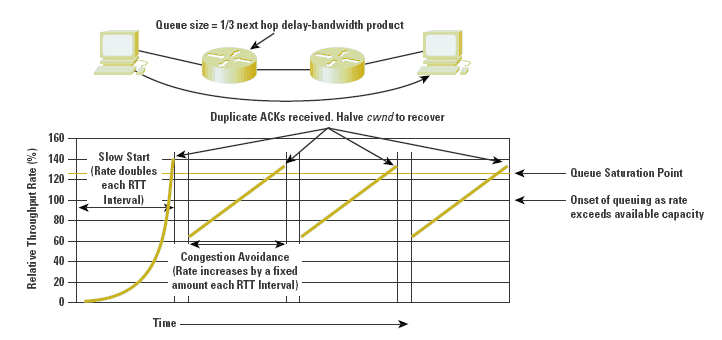Figure 2 - General TCP Rate Control Behaviour

### TCP Slow Start

I always thought this term was somewhat of a misnomer, as its anything but slow once it gets some momentum going! TCP Slow Start mode is used at session startup, and at times when the TCP connection state appears to be been significantly disrupted because of extensive packet loss or periods of connection inactivity. Slow Start is used to quickly establish an initial estimate of the maximal end to end flow rate.

TCP's slow start flow control mechanism is such that the sender increases its sending window by 1 MSS for each received ACK, for as long as the receiver has available advertised buffer space and as long as there are no discarded packets. If the receiver sends an ACK for every packet this would imply a rate doubling every RTT interval. However, most TCP implementations turn on delayed ACK by default, and, generally, these default TCP settings ACK every second packet, implying that TCP will increase the sending rate by an additional 50% every RTT interval.

This is a multiplicative increase that is very sensitive to the round trip time (RTT). For example, if the available path bandwidth is 100Mbps, and the MTU size is 1500 octets and delayed ACKs are in use, then it will take some 10.5 RTT intervals for TCP Slow Start to achieve this path bandwidth. For a LAN with an RTT of less than 1millisecond slow start can reach this bandwidth within 1 or 2 milliseconds. But when the RTT stretches to transcontinental levels of around 30msec then this can take one third of a second in total time, and inter-continental paths of 300msec RTT imply a slow start period of 3 seconds to reach this peak bandwidth level. If the slow start interval time is directly proportional to the RTT, what's the relationship between slow start efficiency and MTU size?

What effect will varying the MTU size have on the performance of the slow start algorithm?

Let's take an example here, of two systems separated by a 24ms network path, no delayed ACKs and TCP timestamps turned on, with a maximum path bandwidth of 100Mbps and look at the performance of slow start over various packet sizes.

 Time (Secs) MTU=1500IPv4(Mbps) MTU=1480IPv4(Mbps) MTU=1400IPv4(Mbps) MTU=1280IPv4(Mbps) MTU=576IPv4(Mbps) 0.000 0 0 0 0 0 0.024 2.04 2.02 1.91 1.75 0.81 0.048 4.09 4.03 3.82 3.50 1.62 0.072 8.17 8.06 7.64 7.00 3.24 0.096 16.34 16.13 15.27 13.99 6.49 0.120 32.68 32.26 30.55 27.99 12.97 0.144 65.37 64.51 61.10 55.98 25.94 0.168 100.09 100.30 100.24 100.15 51.88 0.192 103.77

It will take 0.192 seconds for an IPv4 connection using a 576 byte MTU to reach the bottleneck capacity of 100Mbps. It will 0.168 seconds, or 7 RTT intervals, for an IPv6 connection sized anywhere between 1280 octets and 1500 octets MTU. The range of time from the smallest MTU to the very common 1500 MTU in this example is just 7/100's of a second.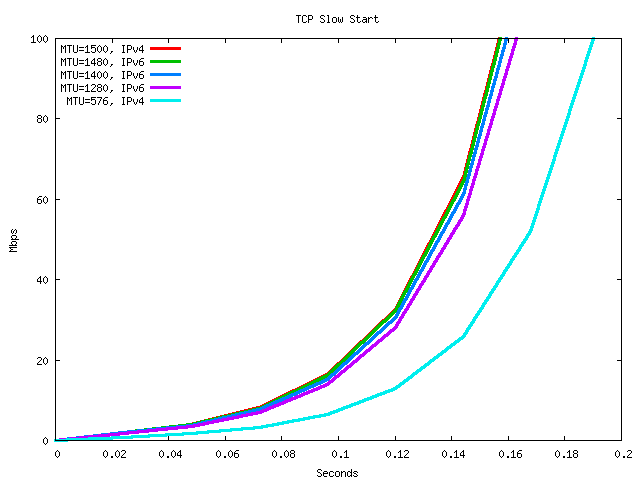Figure 3 - TCP Slow Start

Assuming that the data set to be transferred is large enough, then TCP slow start will continue increasing the sending rate until the sender runs out of local buffer space (as the sender has to keep a copy of all unacknowledged sent data), or the sender thinks the receiver has run out of receiver buffer space (calculated using the receivers advertised buffer size, less the amount of unacknowledged data in flight), the sender reaches a previously cached threshold value for the sending threshold value, of the sender sees an indicated of packet loss through the reception of duplicate ACKs, or the sender's ACK timer expires.

In the first two cases TCP will lock into the buffer-constrained sending rate, and will continue to send at that rate indefinitely, or until at least until either packet loss or ACK timeout occurs. The data rate of this buffer-constrained mode of operation is independent of the MTU size.

In the case of an ACK timeout the session will revert to a restart mode.

In the other cases, TCP will move into Congestion Avoidance mode.

### Buffer Constrained TCP

The receiver advertises its available window with every ACK, and this window limits the amount of additional data that the sender can send into the network before it must stop and wait for a further update with the next ACK.

Similarly the sender operates a local sending buffer that holds all unacknowledged sent data. Once this buffer is filled the sender must stop and await an ACK that clears some data out from this local buffer.

A TCP session operating in a buffer-constrained mode does not have a direct dependency on the MTU size, as the buffer size reflects the amount of unacknowledged data in flight, and not the number of packets used to move the data.

For TCP to operate at maximal performance the sender's buffer and the receiver's buffer must both be greater than the bandwidth delay product of the network path. This is related to the MTU size to some small extent.

In this example, with an available bandwidth of 100Mbps and a round trip delay time of 0.24 seconds, as long as both the receiver and sender have a local buffer of 300,000 octets or greater, then any TCP connection would be capable of operating at maximal speed over the path. To refine this a little, with a maximal TCP data rate of 94.15Mbps with a 1500 octet MTU, the receiver and sender need to have buffers of no less than 282,450 octets. If the MTU is 1400 the maximal data rate is 93.74Mbps and the minimum buffer size of 281,220 octets.

### TCP Congestion Avoidance

TCP's congestion avoidance mode uses a "sawtooth" low frequency oscillation of the sending rate around the estimated sustainable flow rate, constantly probing to see if a higher flow rate can be sustained, and ready to immediately back off once the current flow rate experiences congestion.

This is done by the sender incrementing the value of a sending "congestion window" in response to each received non-duplicate ACK. Within each RTT interval this incremental opening of the congestion window results in an increase in the sending window by a total of 1 MSS.

In response to a packet loss event, signalled by the reception of an ACK packet that indicates a lost packet (a duplicate ACK), then TCP will attempt to repair the loss. In the first instance TCP will wait to see if the duplicate ACK was caused by minor packet reordering. In response to three duplicate ACKs the sender will assume that the cause is network congestion rather than packet reordering, and the sender will repair the packet loss error and halve its current sending rate. Once the loss is repaired TCP will once more start probing higher sending rates by inflating its sending window by a total of 1 MSS unit each RTT time interval, assuming that delayed ACKs are turned off.

This is one area of TCP's performance that is potentially related to MTU size. The congestion rate increase is equivalent to a rate increase per RTT of ((MSS * 8)/RTT)bps In an example configuration with a 24 ms RTT, there will be rate increase of (MSS * 8) / (0.024)**2 bits per second**2.

The following table shows TCP's Congestion Avoidance rate increase using an RTT of 24ms.

 MTU=1500IPv4(Mbps) MTU=1480IPv4(Mbps) MTU=1400IPv4(Mbps) MTU=1280IPv4(Mbps) MTU=576IPv4(Mbps) 20.1 19.6 18.4 16.8 7.3

In this case the rate acceleration of an IPv6 TCP session in congestion avoidance mode, using an MTU of 1280 and an RTT of 24ms is some 16% slower than an IPv4 session using an MTU of 1500 over the same path.

But its not the difference in the rate increase that is critical here. The salient question is: Will a change in the MTU result in a performance change of a TCP session result for TCP in congestion avoidance mode?

In theory, the answer is "No, not really." Assuming that the TCP connection will experience packet loss when the sending rate hits a rate of P bps and TCP then drops to P/2 bps, then rises pack to P bps at a rate of (MTU * 8)/RTT2 repeatedly, then the average sending rate is 3/4 P bps. This average sending rate is independent of the MTU value, as long as the data file is large enough to sustain a number of cycles of the congestion avoidance algorithm.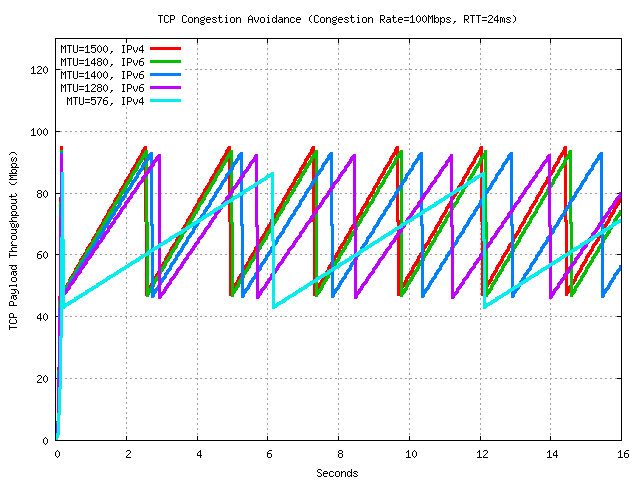Figure 4 - TCP Congestion Avoidance behaviour for a range of MTU settings

Figure 4 shows a simplified data rate for a TCP connection over a connection with a 24ms RTT, using no delayed ACKs where the onset of data loss occurs when the sender reaches a sending rate of 110Mbps. This is simplified in that it is assumed that only a single packet loss occurs when TCP reaches the maximum sending rate, jitter and the effects of RTT lengthening when the network elements' buffer space starts to fill are not included, nor is the TCP recovery behaviour following the loss of the packet included. Note that this figure tracks the effective data rate, and does not include the per-packet overhead of the TCP, IP and Etherframe encapsulation of the data.

The data throughout the results from this performance is shown in Figure 5.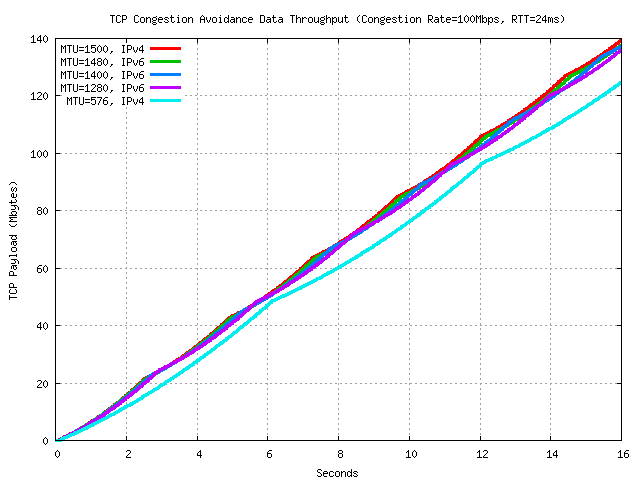Figure 5 - TCP Congestion Avoidance Data Throughput for a range of MTU settings

The variation here in cumulative data throughput is due to the differing per-packet overheads, and not due to any intrinsic difference in the efficiency of TCP in controlling the data flows when the packet size is varied. TCP performance itself is independent of the MTU setting.

### TCP Rate Equation

If TCP performance is really independent of the MTU setting, then why shouldn't we all just use 1280 octet MTU settings on IPv6 hosts, 1500 octet settings on all else and just be done? Why bother with larger packet sizes at all?

There is a common belief that MTU size will impact TCP performance, and that the larger the MTU the higher the TCP performance.

Is there any model of TCP that would confirm this view?

This an extract from "Advice for Internet Subnet Designers" [RFC3819]:

```
8.5.1.  The Formulae

The performance of TCP's AIMD Congestion Avoidance algorithm has been
extensively analyzed.  The current best formula for the performance
of the specific algorithms used by Reno TCP (i.e., the TCP specified
in [RFC2581]) is given by Padhye, et al. [PFTK98].  This formula is:

MSS
BW = --------------------------------------------------------
RTT*sqrt(1.33*p) + RTO*p*[1+32*p^2]*min[1,3*sqrt(.75*p)]

where

BW   is the maximum TCP throughout achievable by an
individual TCP flow
MSS  is the TCP segment size being used by the connection
RTT  is the end-to-end round trip time of the TCP connection
RTO  is the packet timeout (based on RTT)
p    is the packet loss rate for the path
(i.e., .01 if there is 1% packet loss)

Note that the speed of the links making up the Internet path does not
explicitly appear in this formula.  Attempting to send faster than
the slowest link in the path causes the queue to grow at the
transmitter driving the bottleneck.  This increases the RTT, which in
turn reduces the achievable throughput.

This is currently considered to be the best approximate formula for
Reno TCP performance.  A further simplification of this formula is
generally made by assuming that RTO is approximately 5*RTT.

TCP is constantly being improved.  A simpler formula, which gives an
upper bound on the performance of any AIMD algorithm which is likely
to be implemented in TCP in the future, was derived by Ott, et al.
[MSMO97].

MSS   1
BW = C    --- -------
RTT sqrt(p)

where C is 0.93.

8.5.2.  Assumptions

Both formulae assume that the TCP Receiver Window is not limiting the
performance of the connection.  Because the receiver window is
entirely determined by end-hosts, we assume that hosts will maximize
the announced receiver window to maximize their network performance.

Both of these formulae allow BW to become infinite if there is no
loss.  However, an Internet path will drop packets at bottlenecked
queues if the load is too high.  Thus, a completely lossless TCP/IP
network can never occur (unless the network is being underutilized).

The RTT used is the arithmetic average, including queuing delays.

The formulae are for a single TCP connection.  If a path carries many
TCP connections, each will follow the formulae above independently.

The formulae assume long-running TCP connections.  For connections
that are extremely short (<10 packets) and don't lose any packets,
performance is driven by the TCP slow-start algorithm.  For
connections of medium length, where on average only a few segments
are lost, single connection performance will actually be slightly
better than given by the formulae above.

The difference between the simple and complex formulae above is that
the complex formula includes the effects of TCP retransmission
timeouts.  For very low levels of packet loss (significantly less
than 1%), timeouts are unlikely to occur, and the formulae lead to
very similar results.  At higher packet losses (1% and above), the
complex formula gives a more accurate estimate of performance (which
will always be significantly lower than the result from the simple
formula).

Note that these formulae break down as p approaches 100%.

[MSMO97]      Mathis, M., Semke, J., Mahdavi, J. and T. Ott, "The
Macroscopic Behavior of the TCP Congestion Avoidance
Algorithm", Computer Communication Review, Vol. 27,
number 3, July 1997.

[PFTK98]      Padhye, J., Firoiu, V., Towsley, D. and J. Kurose,
"Modeling TCP Throughput: a Simple Model and its
Empirical Validation", UMASS CMPSCI Tech Report TR98-
008, Feb. 1998.

```

It should be noted that this rate equation assumes the operation of delayed ACKs with every second ACK suppressed by the receiver. The action of delayed ACKs on TCP throughput can be made explicit in a slightly different rate equation, described in RFC3448:

The throughput equation is:

```                                   s
X =  ----------------------------------------------------------
R*sqrt(2*b*p/3) + (t_RTO * (3*sqrt(3*b*p/8) * p * (1+32*p^2)))

```

Where:

X is the transmit rate in bytes/second.

s is the packet size in bytes.

R is the round trip time in seconds.

p is the loss event rate, between 0 and 1.0, of the number of loss events as a fraction of the number of packets transmitted.

t_RTO is the TCP retransmission timeout value in seconds.

b is the number of packets acknowledged by a single TCP acknowledgement.

This analysis suggests that TCP performance is directly proportional to the MTU size, and that, for example, doubling the MTU size would double the effective TCP performance, all other things being equal.

But are all other things equal in such a picture?

The other part of this rate equation is that TCP performance is inversely proportional to the square root of the packet loss ratio. If the network loss characteristics are such that large packets have the same probability of loss as smaller packets, then larger packets will yield improved performance.

But packet loss probability is not quite so simple. One source of packet loss is that of bit level corruption, most commonly associated with radio systems, but also visible on wireline transmission systems. The probability of bit level corruption in a transmission frame can be modelled as

```        P = 1 - ((1 - BER)(FRAME_SIZE * 8))
```

Or, if the BER value is sufficiently small,

```        P = BER * [FRAME_SIZE * 8]
```

In other words, the BER-constrained model of TCP performance is one where the packet loss ratio is proportional to the MTU, and this would imply that if packet loss is predominately BER-related then TCP performance is proportional to the ratio of MTU / sqrt(MTU).

However, this is not the complete picture of packet loss, as the second source of packet loss is congestion loss in the network's switching elements. Here the packet loss probability is not necessarily an independent function, and as an individual TCP flow rate reaches the path bottleneck rate, the packet loss probability approaches the value of 1.

Lets see what this rate equation can offer in terms of a throughput calculation.

In our example, to achieve a 98Mbps transfer rate between two points separated by a 24 ms RTT delay with a 1500 byte MTU using IPv6 would require a packet loss ratio of 0.000035, or 35 packets per million. An MTU of 1280 using IPv6 with the same packet loss rate would perform at a maximum of 84Mbps, while a 576 byte MTU using IPv4 would perform at 35Mbps.

The following table shows the result of this TCP performance equation for various MTU sizes and packet loss ratios, using a constant RTT of 24msec and turning off Delayed ACKs.

 Loss Ratio MTU=1500IPv4(Mbps) MTU=1480IPv4(Mbps) MTU=1400IPv4(Mbps) MTU=1280IPv4(Mbps) MTU=576IPv4(Mbps) 0.000001 594 578 545 496 207 0.000010 188 183 172 157 65 0.000024 120 117 110 100 42 0.000035 100 98 92 84 35 0.000100 59 58 54 50 21 0.001000 19 18 17 15 6 0.010000 5 5 5 4 2 0.100000 1 1 1 1 0

Is there some way to check this equation against the theoretical TCP rate model?

One way to do this is to make some assumptions about our example network path to see if the theoretical model of TCP congestion avoidance and this rate equation coincides. The major assumption is that the buffer level at the network path saturation point is minimally adequate, and during an RTT when the sender exceeds the 100Mbps bottleneck transmission capacity using congestion avoidance, the network element will drop a single packet.

This implies that for each congestion avoidance cycle of a rate halving and then a rate increase of 1 MSS per RTT there will only be one packet dropped. So what is the packet loss rate predicted by the congestion avoidance model?

Firstly we start with the peak data rate, as calculated by the packet and framing overheads, assuming a network path with an RTT of 24msec, a bottleneck bandwidth of 100Mbps, a TCP session with no delayed ACKS and timestamps enabled.

 MTU=1500IPv4(Mbps) MTU=1480IPv4(Mbps) MTU=1400IPv4(Mbps) MTU=1280IPv4(Mbps) MTU=576IPv4(Mbps) Peak Rate 94.15 92.75 92.66 92.35 91.65

We can then multiply this by the RTT to give the Mbits per RTT interval at the peak sending rate.

 MTU=1500IPv4(Mb/RTT) MTU=1480IPv4(Mb/RTT) MTU=1400IPv4(Mb/RTT) MTU=1280IPv4(Mb/RTT) MTU=576IPv4(Mb/RTT) Peak Rate 2.260 2.226 2.224 2.216 2.200

We can then multiply this by 125000 to give the peak rate in units of bytes per RTT, and then divide this by the data payload size of the packet to give the peak packet rate per RTT

 MTU=1500IPv4(Pkts/RTT) MTU=1480IPv4(Pkts/RTT) MTU=1400IPv4(Pkts/RTT) MTU=1280IPv4(Pkts/RTT) MTU=576IPv4(Pkts/RTT) Peak Rate 195.1 197.6 209.3 229.3 545.5

Once TCP achieves this peak rate it will then halve its sending rate and then build back to the peak rate at a rate of one additional packet per RTT. If the peak packet rate is P packets per RTT, then the complete TCP congestion avoidance cycle will take (P/2+1) RTT intervals to complete, and the total number of packets sent in a single cycle is (P/2+1) * (P * 3/4), and the loss rate is 1 packet per the number of packets sent in a single cycle.

 MTU=1500IPv4(Mbps) MTU=1480IPv4(Mbps) MTU=1400IPv4(Mbps) MTU=1280IPv4(Mbps) MTU=576IPv4(Mbps) Packets percycle 14,415 14,793 16,588 19,867 112,013 Loss Rate 0.000069 0.000068 0.000060 0.000050 0.000009

The Congestion Avoidance model predicts that the sustained data rate is 3/4 of the peak rate. So now we have the associated packet loss ratios, how does this compare to the rates predicted by the TCP rate equation?

 TCP DateRate MTU=1500IPv4(Mbps) MTU=1480IPv4(Mbps) MTU=1400IPv4(Mbps) MTU=1280IPv4(Mbps) MTU=576IPv4(Mbps) Model 70.61 69.56 69.50 69.26 68.74 Equation 70.27 70.21 70.12 69.87 69.20

It appears that a congestion-driven packet loss model can generate TCP rate equation outcomes where the variation in data throughput when the MTU is varied is related predominately to the differences in the relative overheads of the packet and frame headers rather than any intrinsic limitation of TCP.

So the theory appears to be suggesting that changing the MTU size is not going to result in a dramatic difference in outcomes in terms of data throughput for TCP.

If an IPv6 server uses an MTU of 1500 and relies on the correct operation of Path MTU discovery it can achieve a maximal data throughput of some 92.8% of the available path bandwidth. If the server adopts a more conservative position and uses an IPv6 MTU of 1280 octets the throughput efficiency will drop to some 91.6% of the available path bandwidth. To me this appears to be a relatively minor difference in terms of achievable performance.

But all of this so far is theoretical. Perhaps a relevant question now is: How does this theory work in practice?

I'll report on some experimental findings in my next article on this topic.

```

```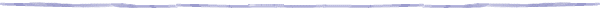### Disclaimer

The above views do not necessarily represent the views of the Asia Pacific Network Information Centre.